# Lines Line Segments and Rays Lines Line Segments

• Slides: 20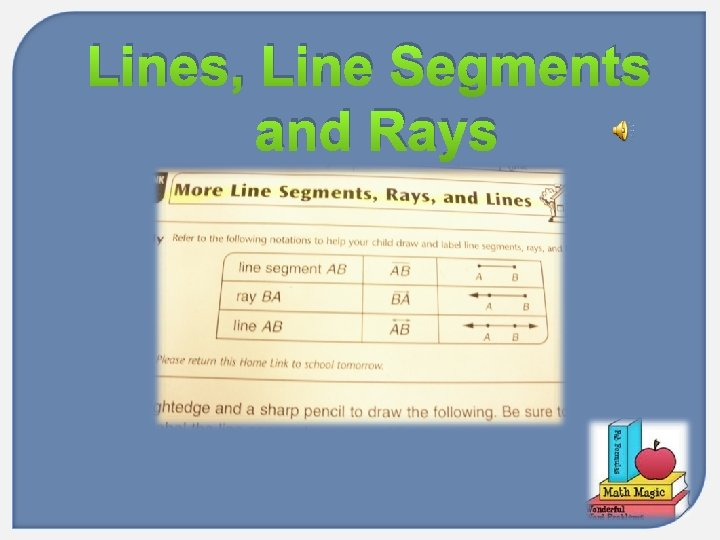Lines, Line Segments and RaysLines, Line Segments and Rays NEW VOCABULARY Line Ray End Point Line Segment Parallel Intersecting Perpendicular MAIN IDEA: To identify, classify and describe lines, line segments and rays.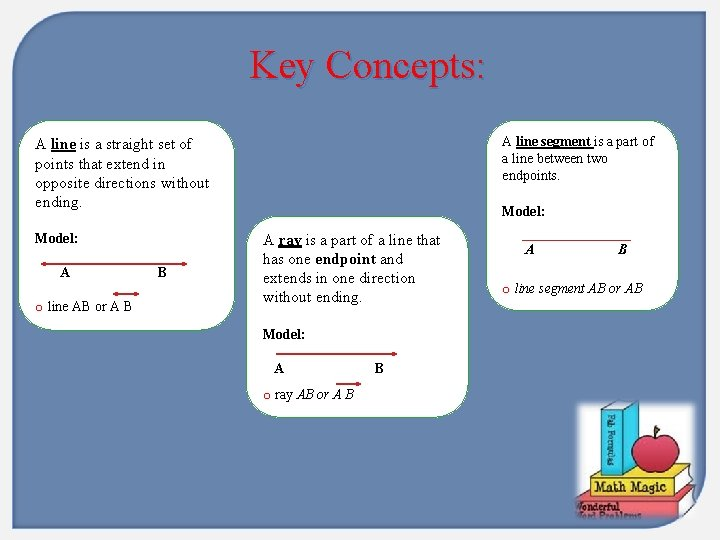Key Concepts: A line segment is a part of a line between two endpoints. A line is a straight set of points that extend in opposite directions without ending. Model: A o line AB or A B B Model: A ray is a part of a line that has one endpoint and extends in one direction without ending. Model: A o ray AB or A B B A B o line segment AB or AB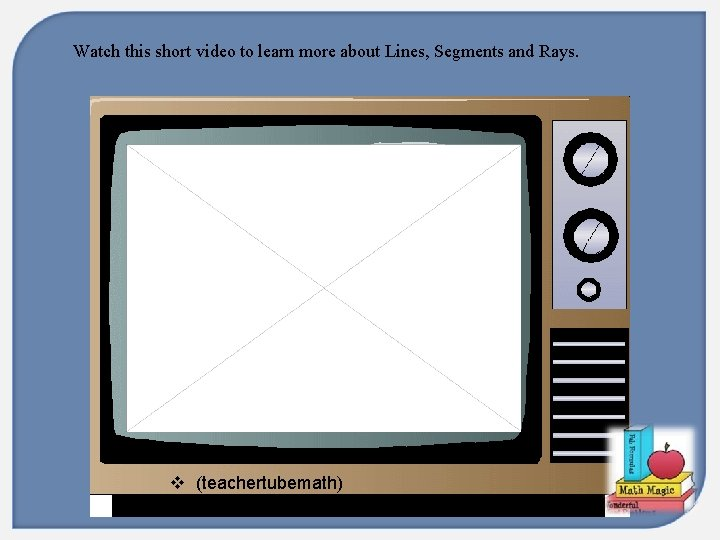Watch this short video to learn more about Lines, Segments and Rays. v (teachertubemath)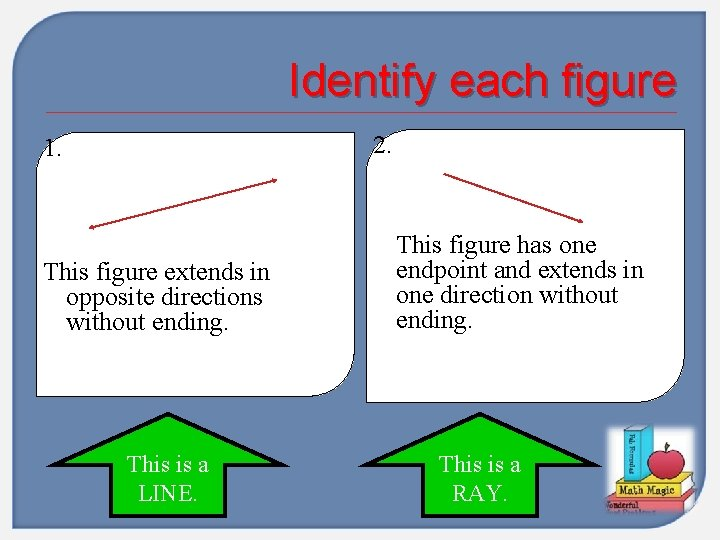Identify each figure 2. 1. This figure extends in opposite directions without ending. This is a LINE. This figure has one endpoint and extends in one direction without ending. This is a RAY.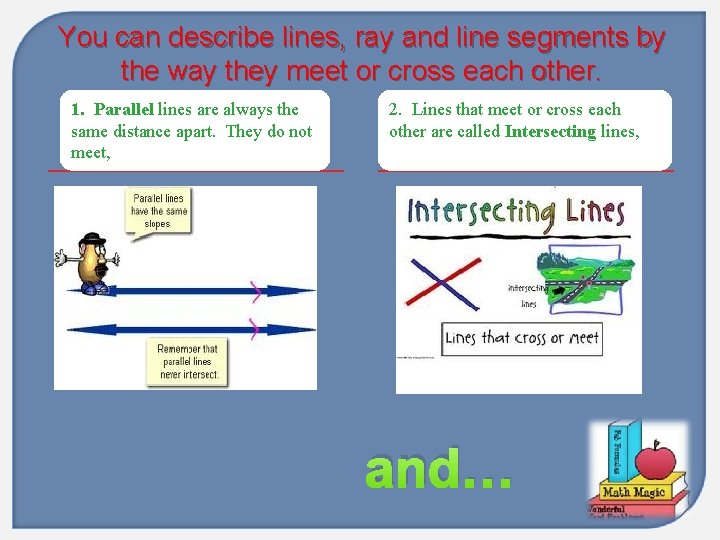You can describe lines, ray and line segments by the way they meet or cross each other. 1. Parallel lines are always the same distance apart. They do not meet, 2. Lines that meet or cross each other are called Intersecting lines, and…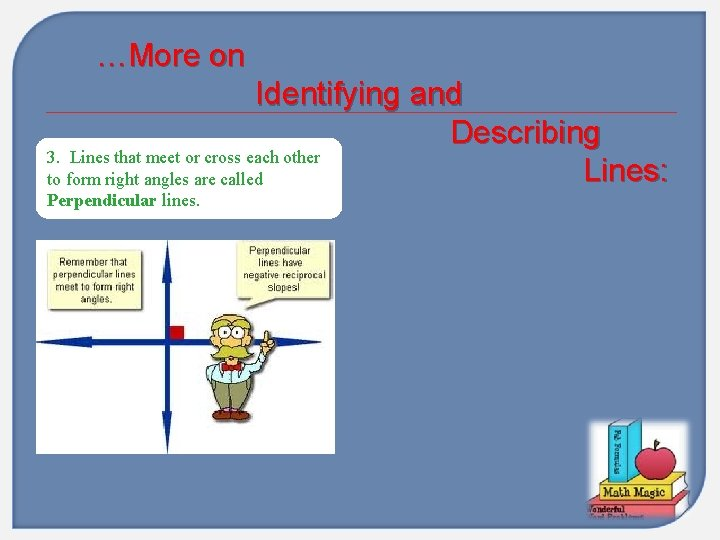…More on Identifying and Describing 3. Lines that meet or cross each other Lines: to form right angles are called Perpendicular lines.Describe the Lines: Describe the figure: The figure shows line JK and line IH. Notice that line JK intersects line IH. JK intersects HI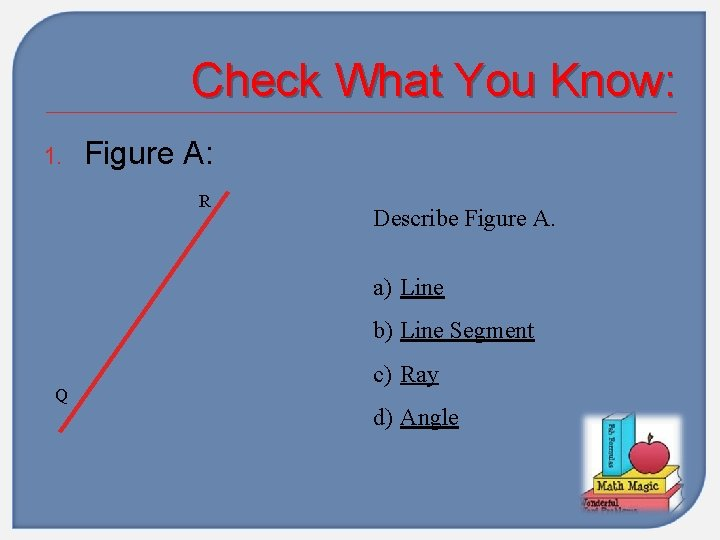Check What You Know: 1. Figure A: R Describe Figure A. a) Line b) Line Segment Q c) Ray d) Angle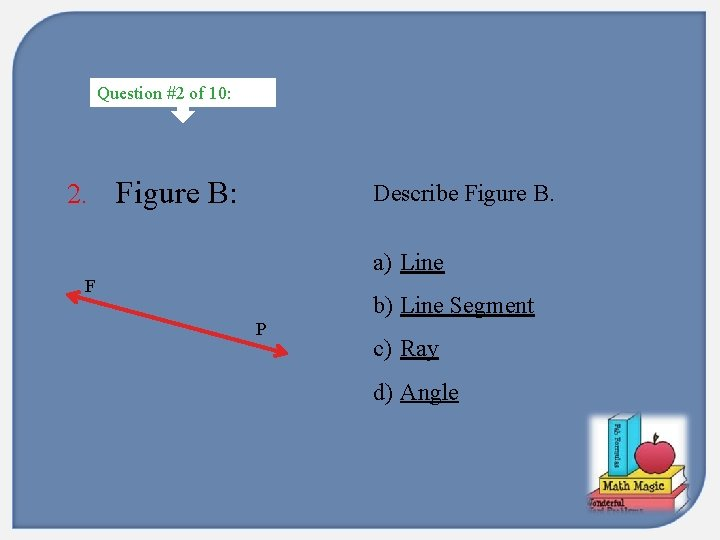Question #2 of 10: 2. Figure B: Describe Figure B. a) Line F b) Line Segment P c) Ray d) Angle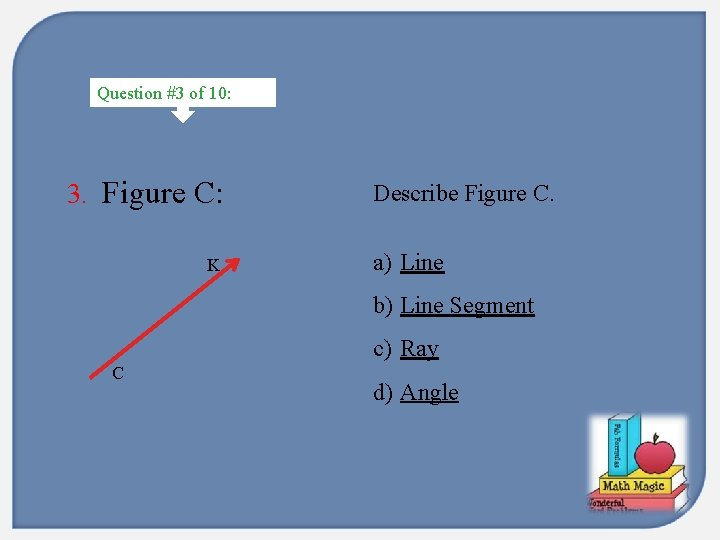Question #3 of 10: 3. Figure C: K Describe Figure C. a) Line b) Line Segment c) Ray C d) Angle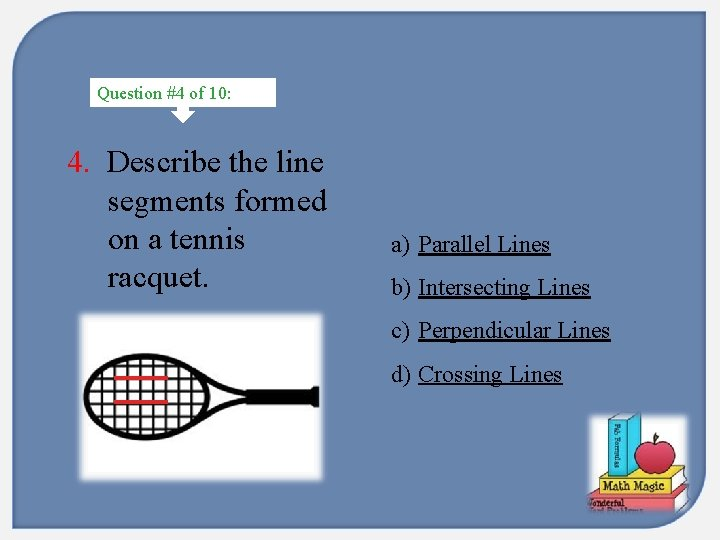Question #4 of 10: 4. Describe the line segments formed on a tennis racquet. a) Parallel Lines b) Intersecting Lines c) Perpendicular Lines d) Crossing Lines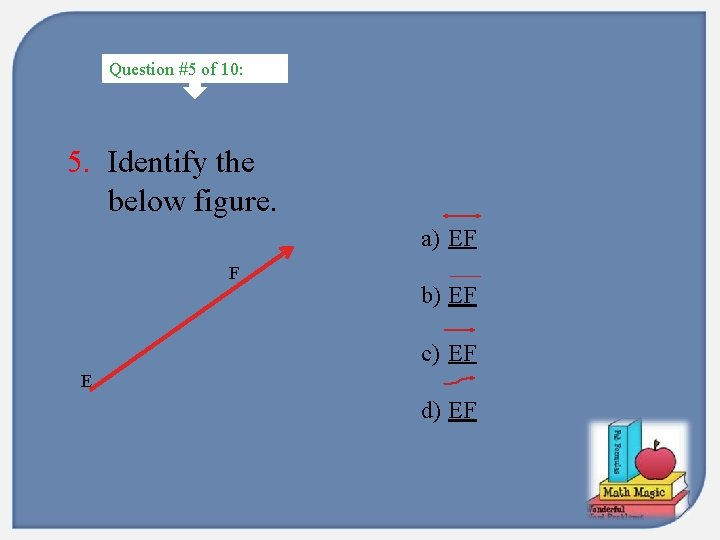Question #5 of 10: 5. Identify the below figure. a) EF F b) EF c) EF E d) EF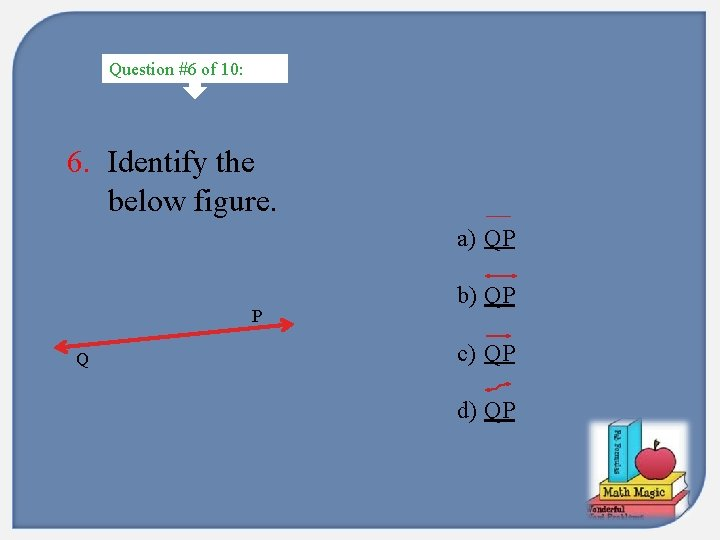Question #6 of 10: 6. Identify the below figure. a) QP P Q b) QP c) QP d) QP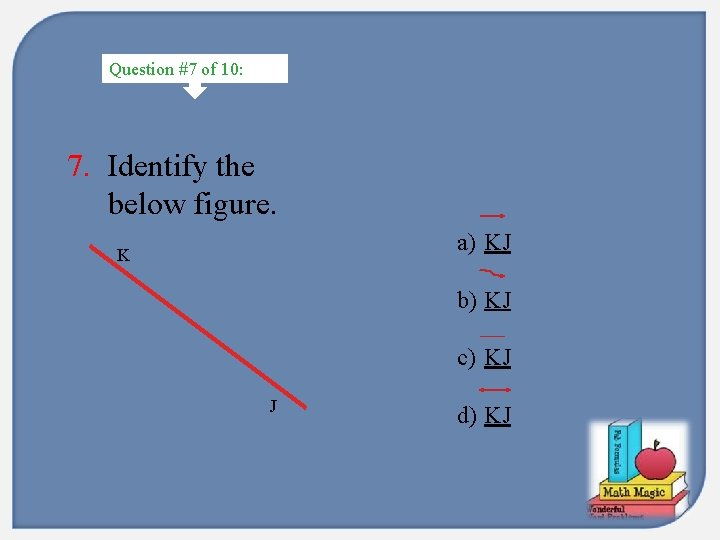Question #7 of 10: 7. Identify the below figure. a) KJ K b) KJ c) KJ J d) KJQuestion #8 of 10: 8. Describe the figure. a) Parallel Lines b) Intersecting Lines c) Perpendicular Lines d) Crossing Lines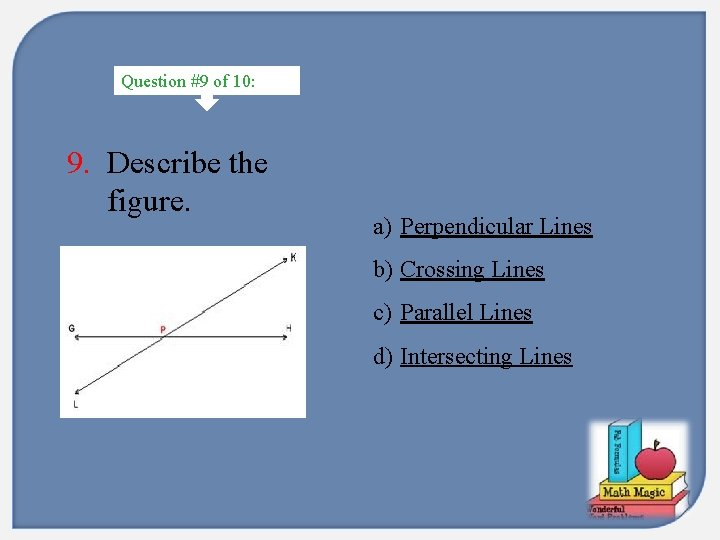Question #9 of 10: 9. Describe the figure. a) Perpendicular Lines b) Crossing Lines c) Parallel Lines d) Intersecting Lines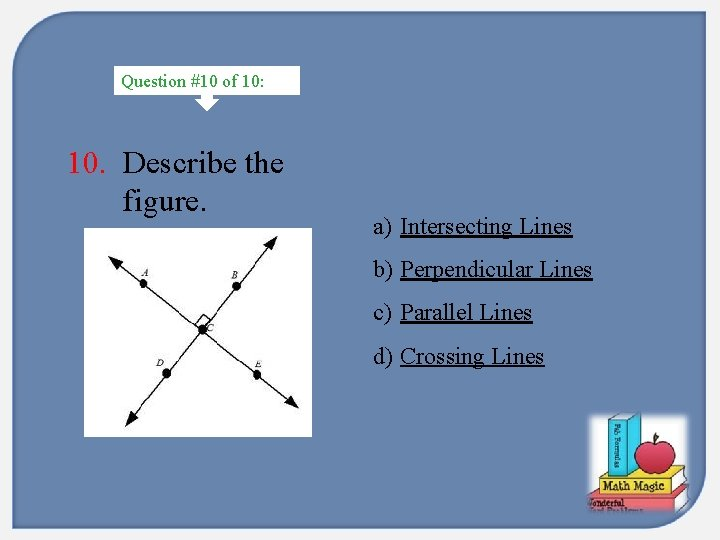Question #10 of 10: 10. Describe the figure. a) Intersecting Lines b) Perpendicular Lines c) Parallel Lines d) Crossing Lines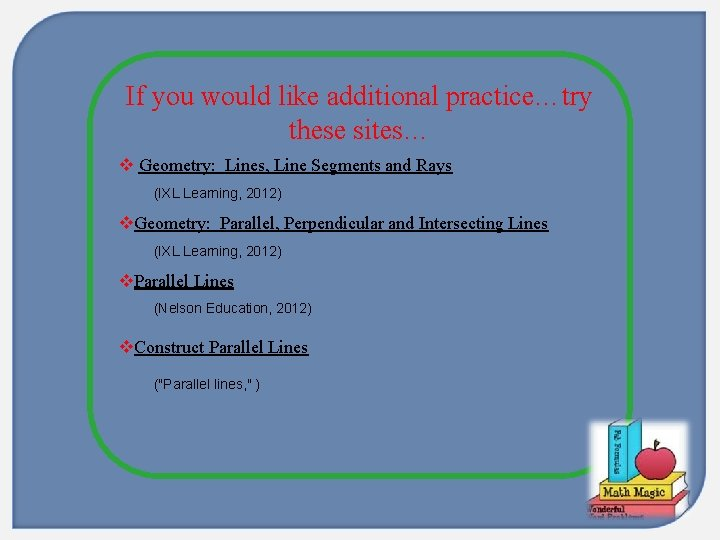If you would like additional practice…try these sites… v Geometry: Lines, Line Segments and Rays (IXL Learning, 2012) v. Geometry: Parallel, Perpendicular and Intersecting Lines (IXL Learning, 2012) v. Parallel Lines (Nelson Education, 2012) v. Construct Parallel Lines ("Parallel lines, " )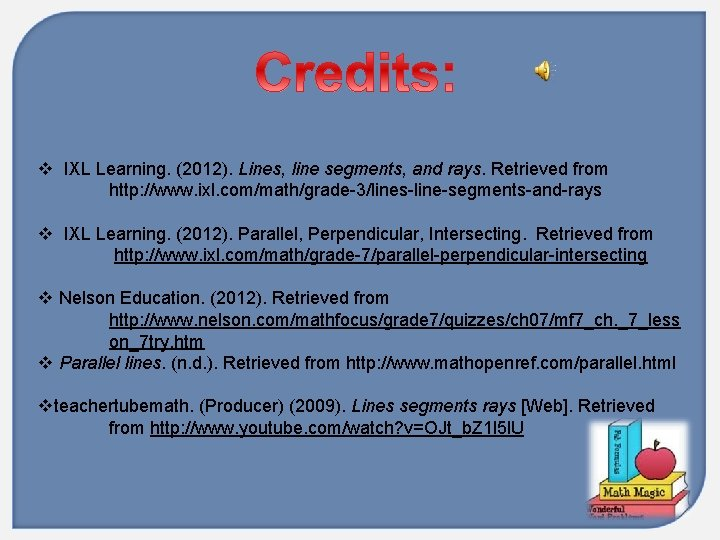v IXL Learning. (2012). Lines, line segments, and rays. Retrieved from http: //www. ixl. com/math/grade-3/lines-line-segments-and-rays v IXL Learning. (2012). Parallel, Perpendicular, Intersecting. Retrieved from http: //www. ixl. com/math/grade-7/parallel-perpendicular-intersecting v Nelson Education. (2012). Retrieved from http: //www. nelson. com/mathfocus/grade 7/quizzes/ch 07/mf 7_ch. _7_less on_7 try. htm v Parallel lines. (n. d. ). Retrieved from http: //www. mathopenref. com/parallel. html vteachertubemath. (Producer) (2009). Lines segments rays [Web]. Retrieved from http: //www. youtube. com/watch? v=OJt_b. Z 1 l 5 l. U